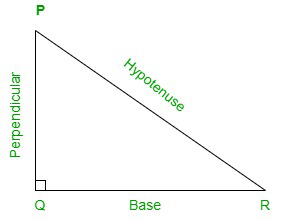GeeksforGeeks App
Open AppBrowser
Continue

# Inverse Tangent Formula

In trigonometry, angles are evaluated with respect to the basic trigonometric functions of trigonometry which are sine, cosine, tangent, cotangent, secant, and cosecant. These trigonometric functions have their own trigonometric ratios under different angles which are used in trigonometric operations. These functions also have their inverses which are known as arcsin, arccos, arctan, arccot, arcsec, and arccosec.

The given article is the study of inverse tangent or arctan. It includes the explanation and derivation of an inverse tangent, inverse tangent formula for the evaluation of angles, and some sample problems.

### What is Inverse Tangent?

Inverse tangent is a function of trigonometry which is an inverse of the trigonometric function tangent. It is also known as the arctan as the prefix ‘-arc’ means inverse in trigonometry. The inverse tangent is denoted by tan-1x.

The inverse tangent function is used to determine the value of the angle by the ratio of (perpendicular/base).

Consider an angle θ and the tangent of the angle equals x. Then, it will give the inverse function of the tangent.

As, x = tanθ

=> θ = tan-1x

Mathematically, the inverse tangent is derived by the ratio of perpendicular by the base.

Let us consider a right-angled triangle PQR.In the right-angled triangle, PQR tangent function will be

=>tan θ = perpendicular/base

θ = tan-1(p/b)

### Inverse Tangent Formula

As tangent is a trigonometric function similarly, the inverse tangent is an inverse trigonometric function of the tangent. The values for these inverse function is derived from the corresponding inverse tangent formula which can either be expressed in degrees or radians.

The list of some of the inverse tangent formulas are given below:

• θ = arctan(perpendicular/base)
• arctan(-x) = -arctan(x) for all x∈ R
• tan(arctan x) = x, for all real numbers
• arctan(1/x) = π/2 – arctan(x) = arccot(x); if x>0

(Or)

• arctan(1/x) = -π/2 – arctan(x) = arccot(x) -π ; if x<0
• sin(arctan x) = x/ √(1+x2)
• cos(arctan x) = 1/ √(1+x2)
• arctan(x) =• arctan(x) =In trigonometry, there is also a separate set of formulas of the inverse tangent with respect to π.

• π/4 = 4 arctan(1/5) – arctan(1/239)
• π/4 =  arctan(1/2) + arctan(1/3)
• π/4 = 2 arctan(1/2) – arctan(1/7)
• π/4 = 2 arctan(1/3) +arctan(1/7)
• π/4 = 8 arctan(1/10) – 4 arctan(1/515) – arctan(1/239)
• π/4 = 3 arctan(1/4) + arctan(1/20) + arctan(1/1985)

### Summarized Table of Inverse Tangent

There are some set standard values for inverse tangent in degrees as well as radians. These values are fixed or derived to make the evaluation of angles even more convenient under the given function. Hence, the below-given table provides these values of inverse tangent in degrees and in radians.

### Sample Problems

Problem 1. Evaluate tan-1(0.577).

Solution:

The value of 0.577 equals  tan30°.

=>0.577=tan(30°)

Then,

=>tan-1(0.577)=tan-1(30°)

=>30°

Problem 2. What is the inverse of tan60°?

Solution:

The value of tan60° equals 1.732.

=>tan60°=1.732

Then,

tan-1(60°)=tan-1(1.732)

=>1.732

Problem 3. What is the inverse of tan45°?

Solution:

The value of tan45° equals 1.

=>tan45°=1

Then,

tan-1(45°)=tan-1(1)

=>1

Problem 4. What is the inverse of tan30°?

Solution:

The value of tan30° equals 0.577

=>tan60°=0.577

Then,

tan-1(30°)=tan-1(0.577)

=>0.577

Problem 5. What is the inverse of tan90°?

Solution:

The value of tan90° equals 0.

=>tan60°=1.732

Then,

tan-1(90°)=tan-1(0)

=>0

My Personal Notes arrow_drop_up
Related Tutorials